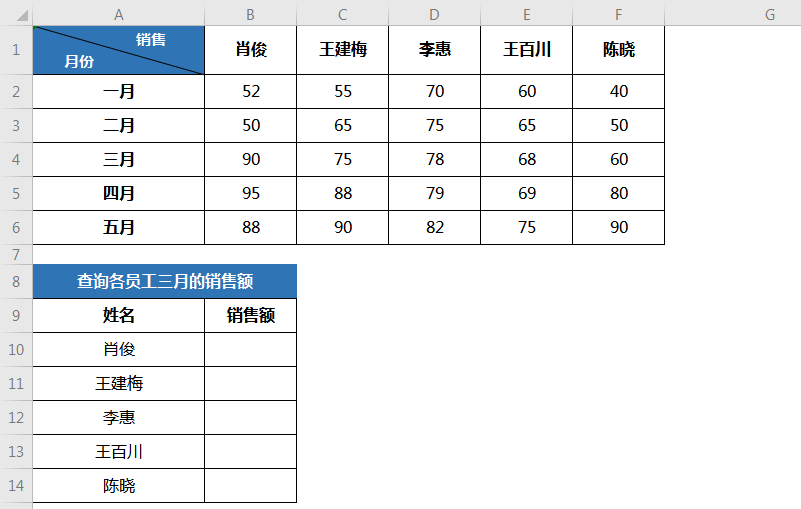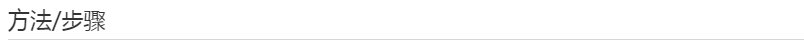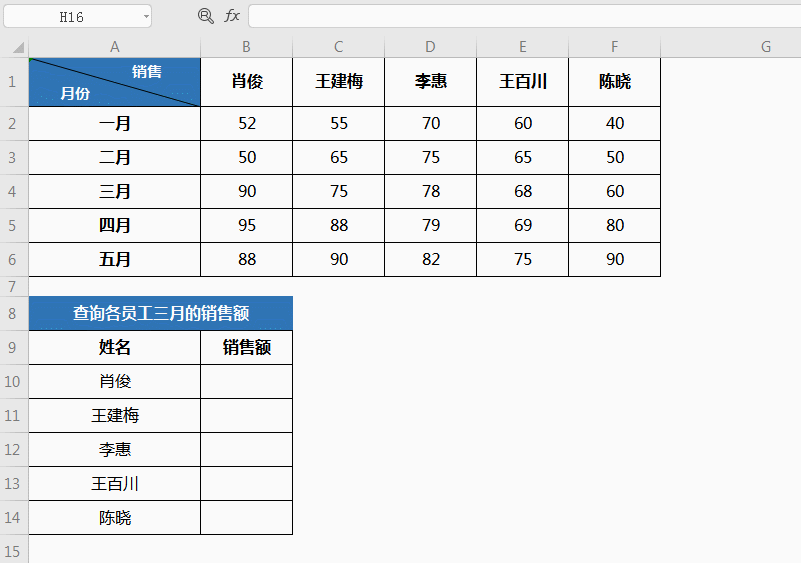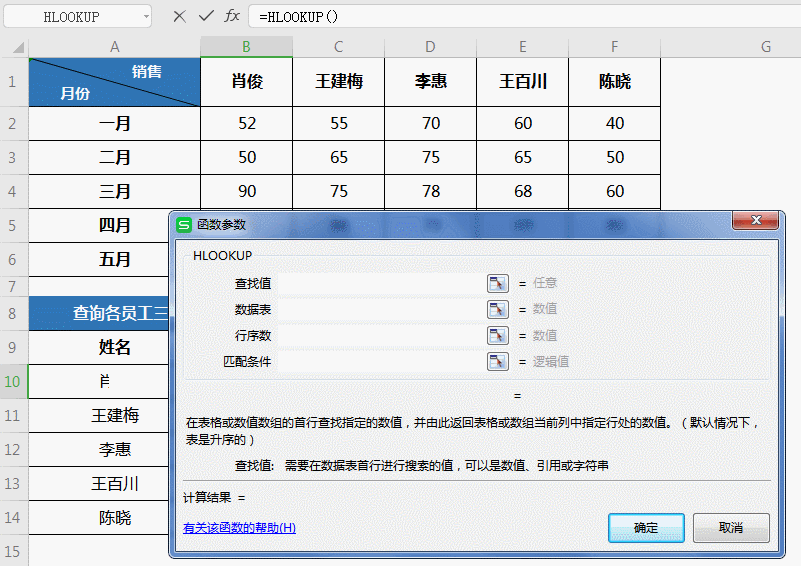## 巧用HLOOKUP横向查找数据

HLOOKUP 中的 H 代表“行”。

HLOOKUP(lookup_value,table_array,row_index_num,range_lookup)

lookup_value 为需要在数据表第一行中进行查找的数值。lookup_value 可以为数值、引用或文本字符串。

Table_array 为需要在其中查找数据的数据表。可以使用对区域或区域名称的引用。

Table_array 的第一行的数值可以为文本、数字或逻辑值。

• 如果 range_lookup 为 TRUE。

• 文本不区分大小写。

• 可以用下面的方法实现数值从左到右的升序排列：

row_index_num 为 table_array 中待返回的匹配值的行序号。

row_index_num 为 1 时，返回 table_array 第一行的数值。

row_index_num 为 2 时，返回 table_array 第二行的数值，以此类推。

Range_LOOKUP 为一逻辑值，指明函数 HLOOKUP 查找时是精确匹配，还是近似匹配。

• 如果函数 HLOOKUP 找不到 lookup_value，且 range_lookup 为 TRUE，则使用小于 lookup_value 的最大值。

• 如果函数 HLOOKUP 小于 table_array 第一行中的最小数值，函数 HLOOKUP 返回错误值 #N/A!。

VLOOKUP的特点是按列查找，可以实现向左向右数据查询、单条件和多条件数据查询等等，功能非常强大。￭选中B10单元格，点击插入函数，在查找框中查找并选中HLOOKUP函数，点击确定；￭此时弹出对话框，共四项参数：